Next: Covariance matrix of experimental Up: Bypassing the Bayes' theorem Previous: Caveat concerning the blind   Contents

# Indirect measurements

Conceptually this is a very simple task in the Bayesian framework, whereas the frequentistic one requires a lot of gymnastics, going back and forth from the logical level of true values to the logical level of estimators. If one accepts that the true values are just random variables6.6, then, calling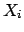a function of other quantities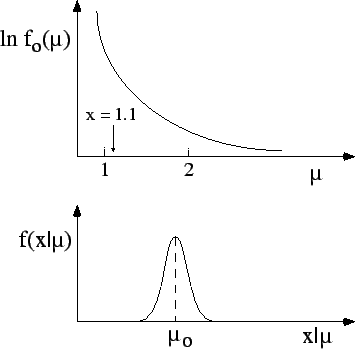, each having a probability density function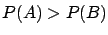, the probability density function of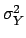can be calculated with the standard formulae which follow from the rules probability. Note that in the approach presented in these notes uncertainties due to systematic effects are treated in the same way as indirect measurements. It is worth repeating that there is no conceptual distinction between various components of the measurement uncertainty. When approximations are sufficient, formulae () and () can be used.

Let us take an example for which the linearization does not give the right result.

Example:
The speed of a proton is measured with a time-of-flight system. Find the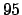,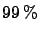and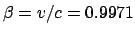probability intervals for the energy, knowing that, and that distance and time have been measured with a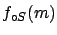accuracy.

The relation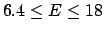is strongly nonlinear. The results given by the approximated method and the correct one are shown in the table below.
 Probability Linearization Correct result (%)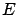(GeV)(GeV) 68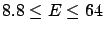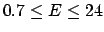95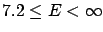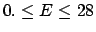99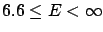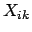Next: Covariance matrix of experimental Up: Bypassing the Bayes' theorem Previous: Caveat concerning the blind   Contents
Giulio D'Agostini 2003-05-15